Publicité

# 541 Interactive ppt Fibonacci Sequence

23 Feb 2014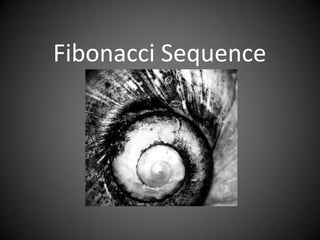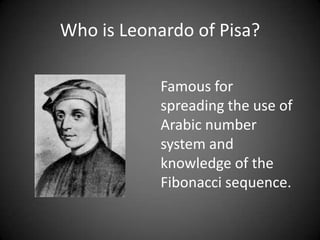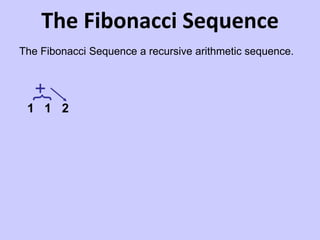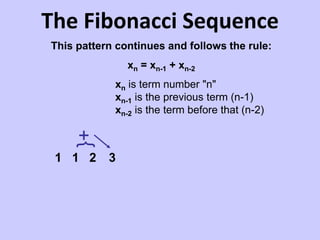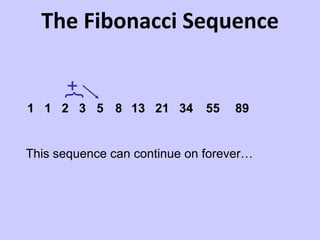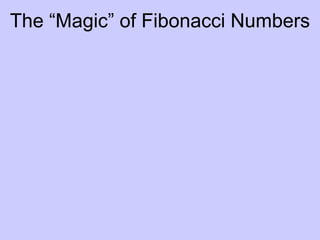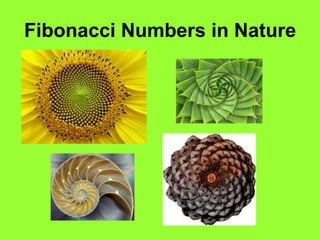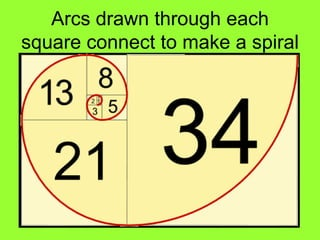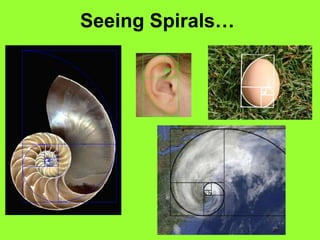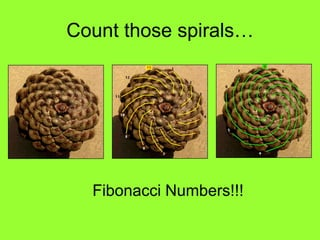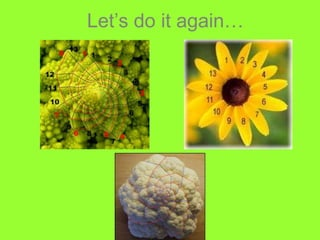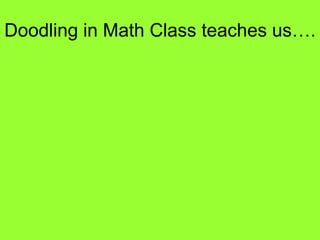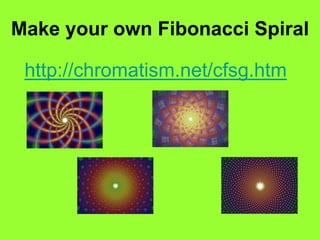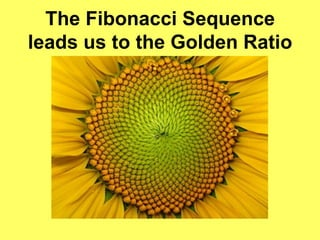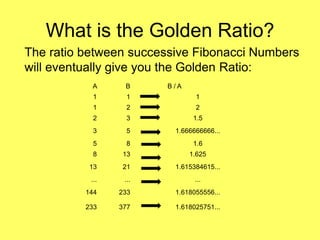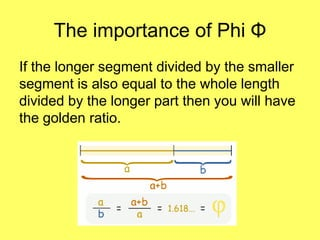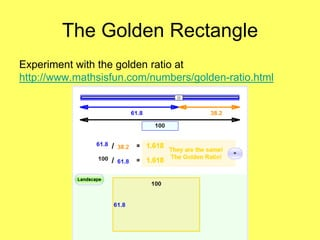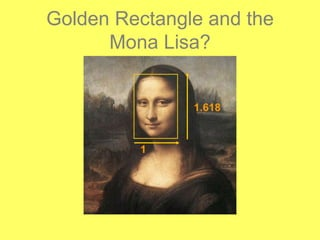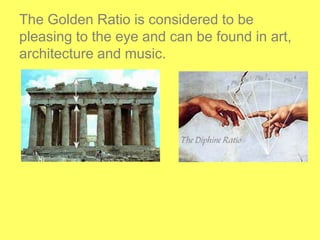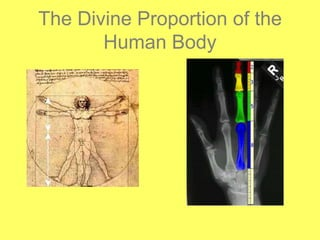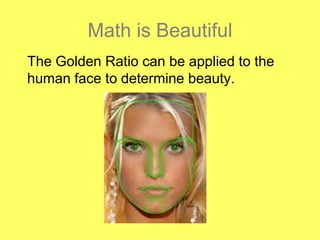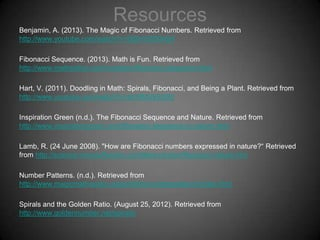1 sur 27
Publicité

### 541 Interactive ppt Fibonacci Sequence

1. Fibonacci Sequence
2. Who is Leonardo of Pisa? Famous for spreading the use of Arabic number system and knowledge of the Fibonacci sequence.
3. The Fibonacci Sequence }+ The Fibonacci Sequence a recursive arithmetic sequence. 1 1 2
4. The Fibonacci Sequence This pattern continues and follows the rule: xn = xn-1 + xn-2 }+ xn is term number "n" xn-1 is the previous term (n-1) xn-2 is the term before that (n-2) 1 1 2 3
5. }+ The Fibonacci Sequence 1 1 2 3 5 8 13 21 34 55 89 This sequence can continue on forever…
6. The “Magic” of Fibonacci Numbers
7. Fibonacci Numbers in Nature
8. Arcs drawn through each square connect to make a spiral
9. Seeing Spirals…
10. Count those spirals… Fibonacci Numbers!!!
11. Let’s do it again…
12. Doodling in Math Class teaches us….
13. Make your own Fibonacci Spiral http://chromatism.net/cfsg.htm
14. The Fibonacci Sequence leads us to the Golden Ratio
15. What is the Golden Ratio? The ratio between successive Fibonacci Numbers will eventually give you the Golden Ratio: A B B/A 1 1 1 1 2 2 2 3 1.5 3 5 1.666666666... 5 8 1.6 8 13 1.625 13 21 1.615384615... ... ... ... 144 233 1.618055556... 233 377 1.618025751...
16. The importance of Phi Φ If the longer segment divided by the smaller segment is also equal to the whole length divided by the longer part then you will have the golden ratio.
17. The Golden Rectangle Experiment with the golden ratio at http://www.mathsisfun.com/numbers/golden-ratio.html
18. Golden Rectangle and the Mona Lisa?
19. The Golden Ratio is considered to be pleasing to the eye and can be found in art, architecture and music.
20. The Divine Proportion of the Human Body
21. Math is Beautiful The Golden Ratio can be applied to the human face to determine beauty.
22. Math of Beauty Interactive According to the Golden Ratio, Jessica Simpson is considered beautiful! Visit this site to participate in a fun activity: http://www.intmath.com/numbers/math-ofbeauty.php